# MG1 Busy Period Power Optimization 1 Power Optimization

• Slides: 11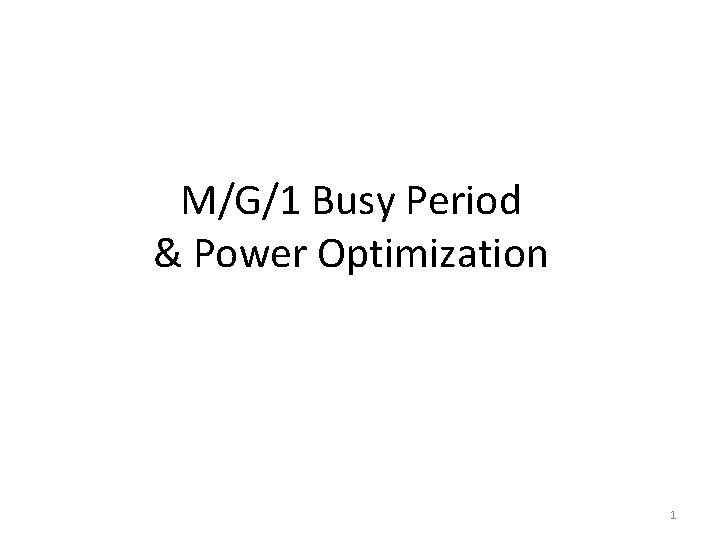M/G/1 Busy Period & Power Optimization 1Power Optimization Problem • Given an M/G/1/FCFS server with the following power consumption profile – – Pon when serving jobs Pidle ≤ Pon when idle waiting for work 0 when off Psetup ≈ Pon when starting up (setup time has general distribution jobs arriving according to a Poisson process of rate λ, and with a general service time distribution • What is the optimal policy that maximizes performance/watt, i. e. , 1/(E[Power] E[T])? 2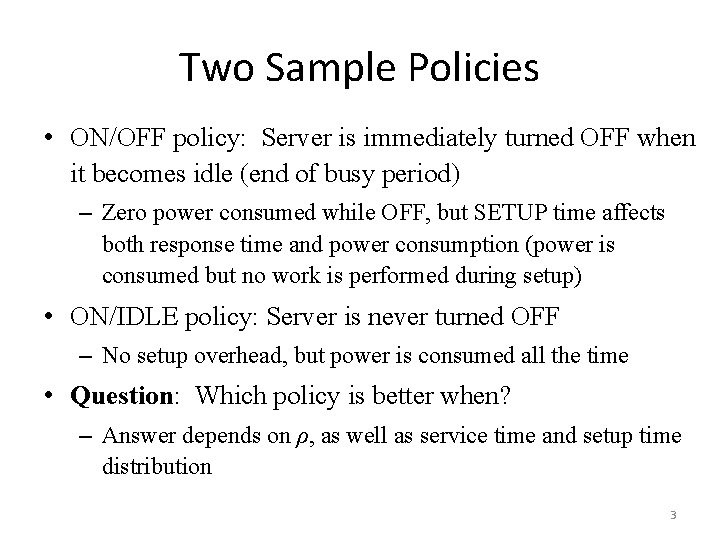Two Sample Policies • ON/OFF policy: Server is immediately turned OFF when it becomes idle (end of busy period) – Zero power consumed while OFF, but SETUP time affects both response time and power consumption (power is consumed but no work is performed during setup) • ON/IDLE policy: Server is never turned OFF – No setup overhead, but power is consumed all the time • Question: Which policy is better when? – Answer depends on ρ, as well as service time and setup time distribution 3M/G/1 Busy Period Analysis – (1) • Busy period distribution plays a major role in the performance of both sample policies • We’ll first derive the Laplace transform Bx(s) of the duration B(x) of a busy period started by an amount of work x, i. e. , B(x) = x +Σ{i=1 to Ax}Bi where the Bi’s are independent busy periods (each started by an arrival) with the same distribution • We then have Bx(s) = e–sx Lf(Σ{i=1 to Ax}Bi) = e–sx Ax(B(s)) = e–sx e–λx(1–B(s)) = e–x(s+λ–λB(s)) • Unconditioning then yields the following expression B(s) = {x=0 to }Bx(s)f. S(x)dx = {x=0 to } e–x(s+λ–λB(s)) f. S(x)dx = S(s+λ–λB(s)) – a recursive expression from which we can derive all moments, including E[B] = E[S]/(1–ρ) 4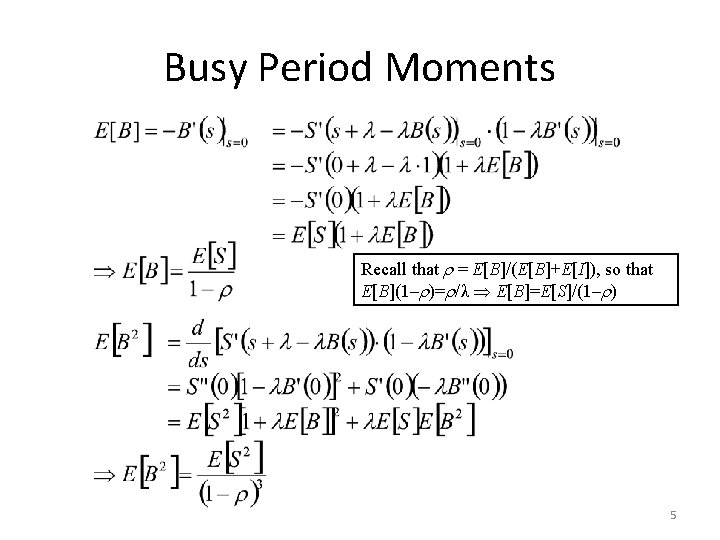Busy Period Moments Recall that = E[B]/(E[B]+E[I]), so that E[B](1– )= /λ E[B]=E[S]/(1– ) 5Busy Period vs. Response Time • Comparing E[B] to E[T], we see that – E[B] = E[S]/(1–ρ) and E[T] = λE[S 2]/2(1–ρ) + E[S] In other words, E[B] is not affected by variability in S, while E[T] is – This because Busy periods start with no jobs in the system, i. e. , they do not depend on Se • In general, the ith moment of B depends on the ith moment of S, while the ith moment of T depends on the (i+1)th moment of S 6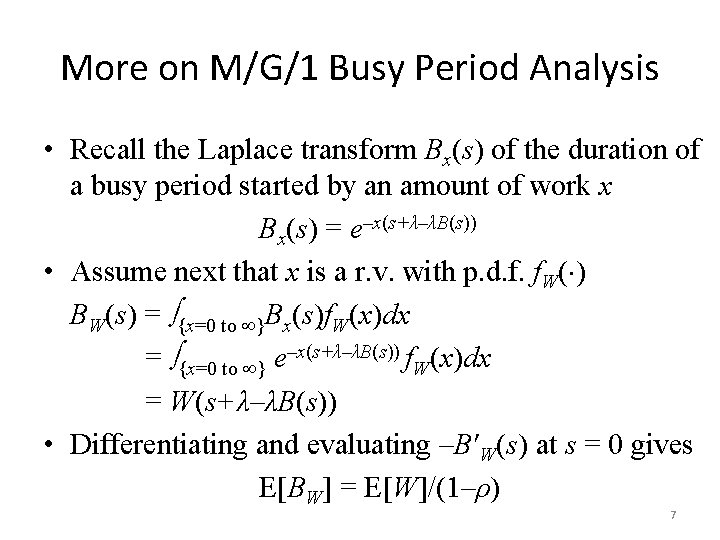More on M/G/1 Busy Period Analysis • Recall the Laplace transform Bx(s) of the duration of a busy period started by an amount of work x Bx(s) = e–x(s+λ–λB(s)) • Assume next that x is a r. v. with p. d. f. f. W( ) BW(s) = {x=0 to }Bx(s)f. W(x)dx = {x=0 to } e–x(s+λ–λB(s)) f. W(x)dx = W(s+λ–λB(s)) • Differentiating and evaluating –B W(s) at s = 0 gives E[BW] = E[W]/(1–ρ) 7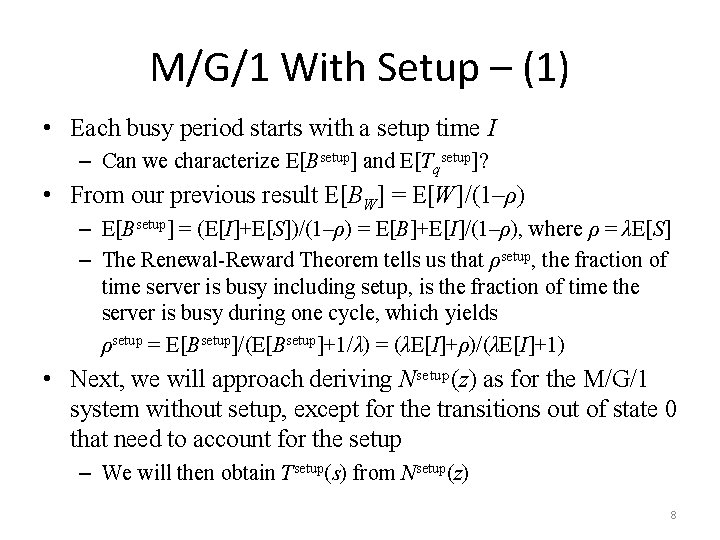M/G/1 With Setup – (1) • Each busy period starts with a setup time I – Can we characterize E[Bsetup] and E[Tqsetup]? • From our previous result E[BW] = E[W]/(1–ρ) – E[Bsetup] = (E[I]+E[S])/(1–ρ) = E[B]+E[I]/(1–ρ), where ρ = λE[S] – The Renewal-Reward Theorem tells us that ρsetup, the fraction of time server is busy including setup, is the fraction of time the server is busy during one cycle, which yields ρsetup = E[Bsetup]/(E[Bsetup]+1/λ) = (λE[I]+ρ)/(λE[I]+1) • Next, we will approach deriving Nsetup(z) as for the M/G/1 system without setup, except for the transitions out of state 0 that need to account for the setup – We will then obtain Tsetup(s) from Nsetup(z) 8M/G/1 With Setup – (2) • Let aj = P{j arrivals in S} – in service time a'j = P{j arrivals in S+I} – in service+setup time • Then Pij = aj-i+1 for i>0 and P 0 j = a'j • Thus πj = π0 a'j + Σ{i=1 to j+1}πiaj-i+1 and – Nsetup(z) = π0 AS+I(z) + 1/z[Nsetup(z) – π0]AS(z) Nsetup(z) = π0[z. AS(z) AI(z) – AS(z)]/[z – AS(z)] where π0 = 1–ρsetup = (1–λE[S])/(1+λE[I]) • We can now obtain Tsetup(s) from Nsetup(z) as before 9M/G/1 With Setup – (3) • • Recall that we have AS(z) = S(λ(1–z)) for any S This implies ATsetup (z) = Tsetup(λ(1–z)), an hence Nsetup(z) = Tsetup(λ(1–z)) since ATsetup (z) = Nsetup(z) • From our previous result, we have • Tsetup(λ(1–z)) = π0[z. AS(z) AI(z) – AS(z)]/[z – AS(z)] = π0[z. S(λ(1–z))I(λ(1–z))–S(λ(1–z))]/[z–S(λ(1–z))] with π0 = 1–ρsetup = (1–λE[S])/(1+λE[I]) = (1–ρ)/(1+λE[I]) Tsetup(s) = π0[(λ–s)S(s)I(s) – λS(s)]/[λ–s–λS(s)], where again s=λ(1–z) • This also gives TQsetup(s) = Tsetup(s)/S(s) and therefore TQsetup(s) = π0[λ–(λ–s)I(s)]/[s –λ+λS(s)], from which we derive TQsetup(s) = TQM/G/1(s) [λ–(λ–s)I(s)]/[s(1+λE[I])] – recall TQM/G/1(s)=(1–ρ)s/[s –λ+λS(s)] • Differentiating yields E[TQsetup] = λE[S 2]/2(1–ρ)+(2 E[I]+λE[I 2])/2(1+λE[I]) If I is exponential E[I 2]=2 E[I]2 so that E[TQsetup] = E[TQ] + E[I] 10Comparing ON/IDLE vs. ON/OFF • ON/IDLE – E[Power]ON/IDLE = ρPon+(1–ρ)Pidle – E[T]ON/IDLE = λE[S 2]/2(1–ρ)+E[S], where ρ = λE[S] • ON/OFF – E[Power]ON/OFF = ρsetup Pon= Pon(λE[I]+ρ)/(λE[I]+1) – E[T]ON/OFF = λE[S 2]/2(1–ρ)+(2 E[I]+λE[I 2])/2(1+λE[I])+E[S] • Performance metric: 1/(E[Power] E[T]) – performance per Watt EXAMPLE • Pon = 240 W and Pidle = 180 W • E[S] = 1 and E[S 2] = 20 • E[I] varies from 0. 125 to 8 • E[I 2] = 5 E[I] • ρ varies from 0. 01 to 0. 99 • At low load ON/OFF is clearly much better when the setup time is low, but this quickly reverses when the setup increases At high load, both policies are equivalent as the server is busy most of the time • 11